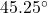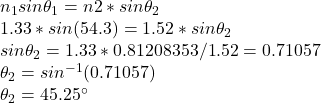A light ray moving at a 54.3 deg angle in water (n = 1.33) hits a boundary with oil (n = 1.52). At what angle is it refracted

Question

A light ray moving at a 54.3 deg
angle in water (n = 1.33) hits a
boundary with oil (n = 1.52). At
what angle is it refracted in the oil?
(Water n = 1.33, Air n = 1.00)
(Unit = deg)​

in progress 0
5 months 2021-08-27T15:18:58+00:00 1 Answers 0 views 0